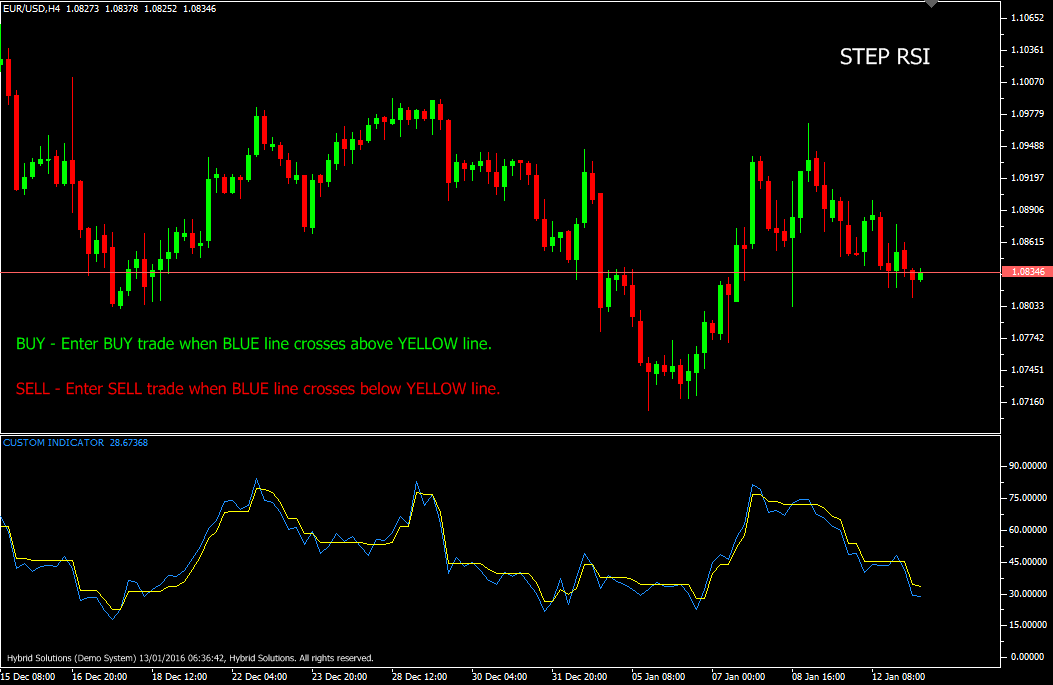# Step RSI

Free

Developed By: NeeduVerdeSolution

In stock

Step RSI is an innovative Vertex FX client side VTL indicator based upon the combination of Relative Strength Index (RSI) and Simple Moving Average (SMA). It provides signals based upon the RSI crossover, and should be used in combination with other trending indicators.In the first step, we calculate the Relative Strength Index (RSI) based upon the PRICE FIELD and RSI METHOD.

SKU: 925 Categories: ,Step RSI is an innovative Vertex FX client side VTL indicator based upon the combination of Relative Strength Index (RSI) and Simple Moving Average (SMA). It provides signals based upon the RSI crossover, and should be used in combination with other trending indicators.In the first step, we calculate the Relative Strength Index (RSI) based upon the PRICE FIELD and RSI METHOD.

In the second step, we calculate the Simple Moving Average (SMA) of the PRICE FIELD based upon the MA PERIOD. In the third step, we calculate the Relative Strength Index (RSI) of the Simple Moving Average (SMA) calculated in the second step. Finally, the Step RSI is calculated from the previous step. Here, we calculate the Relative Strength Index (RSI) in steps of STEP SIZE. The BLUE line is the Relative Strength index calculated in the third step. The YELLOW line is the Simple Moving Average of the Relative Strength Index (RSI) calculated in the third step, and calibrated to increase or decrease in STEP SIZE.

Buy: Place Buy trade when BLUE line crosses above YELLOW line. Use other trending indicators in addition to this indicator for reference. Do not enter BUY trade if candle has gap-up opening. Place Stop-loss below nearest Swing Low.

Sell: Place Sell trade when BLUE line crosses below YELLOW line. Use other trending indicators in addition to this indicator for reference. Do not enter SELL trade if candle has gap-down opening. Place Stop-loss above nearest Swing High.